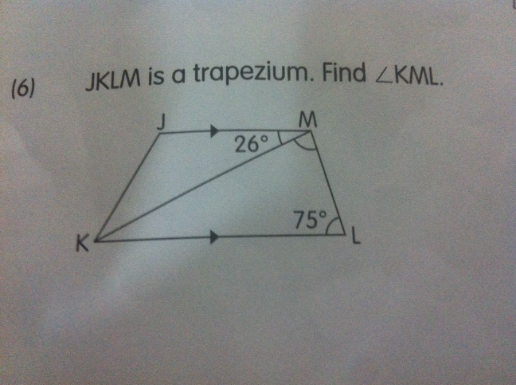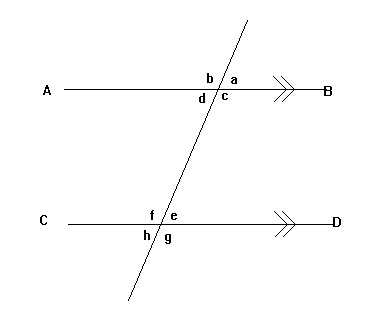# QuestionPlease help thanks

3 Answers

# Answer

angle MKL = angle JMK = 26º

angle KML = 180 – 26 – 75 = 79º

Ans : 79º.

0 Replies 1 Like ✔Accepted Answer

Well, you explain many things in the right way and teaching us for better understanding. The paper writing services share some of the latest book guides which help us a lot in the right way.

0 Replies 0 LikesHi Nav,

I think it is important for your child to memorise the properties of angles related to parallel lines.

With reference to the diagram above,

Corresponding angles

∠a = ∠e ;  ∠b = ∠f ;  ∠d = ∠h ;  ∠c = ∠g

Alternate angles

∠d = ∠e ;  ∠c = ∠ f

Sum of angles between parallel lines = 180º

∠c + ∠e = 180º

∠d + ∠f = 180º

1 Reply 1 Like